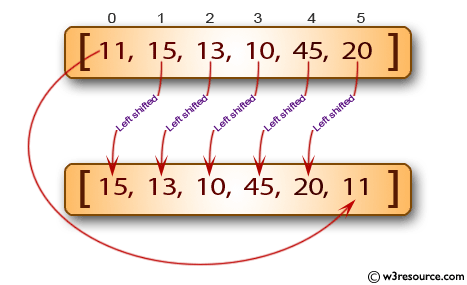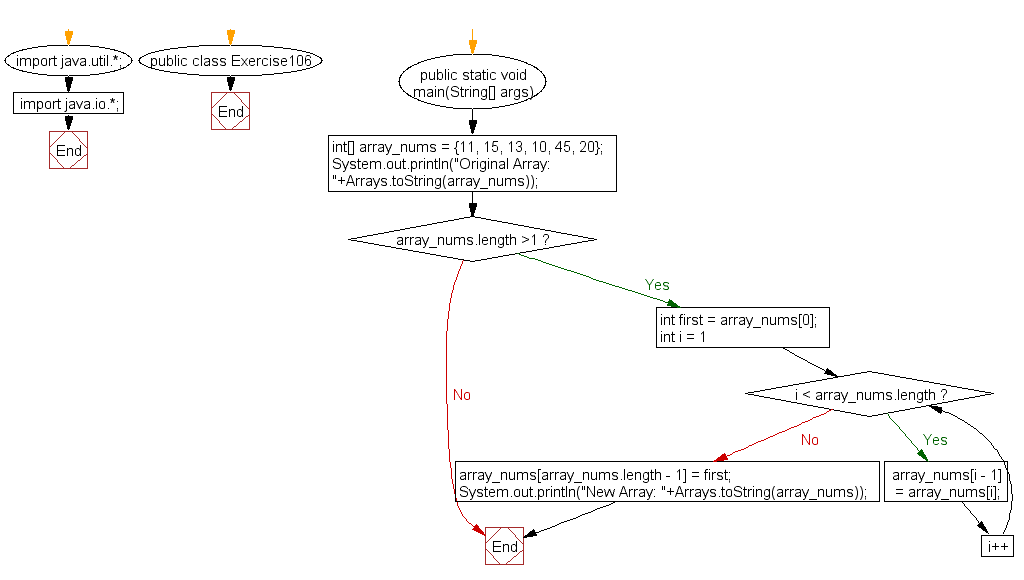﻿ Java exercises: Create a new array that is left shifted from a given array of integers - w3resource# Java Exercises: Create a new array that is left shifted from a given array of integers

## Java Basic: Exercise-106 with Solution

Write a Java program to create a new array that is left shifted from a given array of integers.

Pictorial Presentation:Sample Solution:

Java Code:

``````import java.util.*;
import java.io.*;
public class Exercise106 {
public static void main(String[] args)
{
int[] array_nums = {11, 15, 13, 10, 45, 20};
System.out.println("Original Array: "+Arrays.toString(array_nums));

if(array_nums.length >1)
{
int first = array_nums;

for(int i = 1; i < array_nums.length; i++)
array_nums[i - 1] = array_nums[i];

array_nums[array_nums.length - 1] = first;
System.out.println("New Array: "+Arrays.toString(array_nums));
}
}
}
```
```

Sample Output:

```Original Array: [11, 15, 13, 10, 45, 20]
New Array: [15, 13, 10, 45, 20, 11]
```

Flowchart:Java Code Editor:

What is the difficulty level of this exercise?

﻿

## Java: Tips of the Day

Execution of console commands:

Java allows you to execute console commands directly from code using a class Runtime. It is very important not to forget about exception handling.

For example, let's try to open a PDF file through a Java terminal:

```import java.io.BufferedReader;
import java.io.InputStream;

public class ShellCommandExec {

public static void main(String[] args) {
String gnomeOpenCommand = "gnome-open //home//user//Documents//MyDoc.pdf";

try {
Runtime rt = Runtime.getRuntime();
Process processObj = rt.exec(gnomeOpenCommand);

InputStream stdin = processObj.getErrorStream();

String myoutput = "";